# Riemann xi-function

(diff) ← Older revision | Latest revision (diff) | Newer revision → (diff)-function

In 1859, the newly elected member of the Berlin Academy of Sciences, B.G. Riemann published an epoch-making nine-page paper [a5] (see also [a1], p. 299). In this masterpiece, Riemann's primary goal was to estimate the number of primes less than a given number (cf. also de la Vallée-Poussin theorem). Riemann considers the Euler zeta-function (also called the Riemann zeta-function or Zeta-function)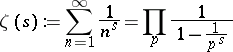(a1)

for complex values of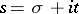, where the product extends over all prime numbers and the Dirichlet series in (a1) converges for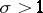(cf. also Zeta-function). His investigation leads him to define a function, called the Riemann-function,(a2)

wheredenotes the gamma-function. The function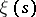is a real entire function of order one and of maximal type and satisfies the functional equation[a6], p. 16. By the Hadamard factorization theorem (cf. also Hadamard theorem),whereranges over the roots of the equation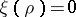. These roots (that is, the zeros of the Riemann-function) lie in the strip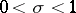. The celebrated Riemann hypothesis (one of the most important unsolved problems in mathematics as of 2000) asserts that all the roots oflie on the critical line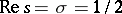(cf. [a2], [a1], [a3], [a6]; cf. also Riemann hypotheses).

The appellation "Riemann x-function" is also used in reference to the function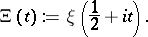(In [a5], Riemann uses the symbolto denote the function which today is denoted by.) In fact, Riemann states his conjecture in terms of the zeros of the Fourier transform [a4], p. 11,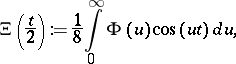whereThe Riemann hypothesis is equivalent to the statement that all the zeros ofare real (cf. [a6], p. 255). Indeed, Riemann writes "[…] es ist sehr wahrscheinlich, dass alle Wurzeln reell sind." (That is, it is very likely that all the roots of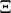are real.)

How to Cite This Entry:
Riemann xi-function. Encyclopedia of Mathematics. URL: http://encyclopediaofmath.org/index.php?title=Riemann_xi-function&oldid=16941
This article was adapted from an original article by George Csordas (originator), which appeared in Encyclopedia of Mathematics - ISBN 1402006098. See original article